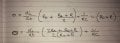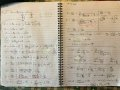# Combining equations inside brackets addition

Thread Starter

#### KevinEamon

Joined Apr 9, 2017
281
Hi guys;

I've been butting my head up against something for several hours.

This is probably the easiest thing in the world for some of you, but I just can't see past it.
The following is part of an equationNow that's fine - I'm sure it all works out nicely, but how is the problem.
I can learn this by memory and it's fine, but I'd like to understand the process of what's happening here.

Can someone give me and intermediate step? I'm trying all sorts of stuff - cross multiplications dividing the whole lot by the R0 + R.

I've got close to the next line
2R0 + 2R0/ 2(R0+R)
but that's not correct according to the solution

#### Papabravo

Joined Feb 24, 2006
15,504
The first step is easy, cross multiply and put the result over the product of the denominators.

$\large R_0\;+\;\frac{R_0\;+\;R}{2}\;=\;\frac{2R_0\;+\;R_0\;+\;R}{2}$

Dividing the result of step 1 by (R₀+R) is the same as multiplication by the reciprocal.

$\large \frac{2R_0\;+\;R_0\;+\;R}{2} \cdot \frac{1}{R_0\;+\;R}\;=\; \frac{2R_0\;+\;R_0\;+\;R}{2(R_0\;+\;R)}$

It's just Algebra -- nothing to remember except basic operations.

Last edited:
•KevinEamon
Thread Starter

#### KevinEamon

Joined Apr 9, 2017
281
You're a legend Papa I couldn't see the forest for the trees...

#### Papabravo

Joined Feb 24, 2006
15,504
•KevinEamon
Thread Starter

#### KevinEamon

Joined Apr 9, 2017
281
Lol... well that makes two of us, two more and we can play a four ball#### WBahn

Joined Mar 31, 2012
26,398
Can someone give me and intermediate step? I'm trying all sorts of stuff - cross multiplications dividing the whole lot by the R0 + R.
If you show us your actual attempt, we could point out the specific point where you are going wrong.

I've got close to the next line
2R0 + 2R0/ 2(R0+R)
but that's not correct according to the solution
You KNOW that this isn't correct because of the units. Your first term is 2R0, so it has units of resistance. Your second term simpifies to R0(R0+R) and so has units of resistance-squared.

This is probably not what you meant, but this is what you wrote. You need to pay close attention to order of operations, particularly give your weak algebra skills. This has caused you lots of grief in the past and will continue to do so.

What you wrote is equivalent to

(2R0) + (((2R0)/2)(R0+R))

What I think you likely meant was

(2R0) + ((2R0) / (2(R0+R)))

which also has units issues since it is now a resistance added to a dimensionless quantity.

Or perhaps you meant

((2R0) + (2R0))/(2(R0+R))

While this is dimensionally consistent, I'm guessing it's not what you meant since I would have expected you to have written it as simply

2R0/(R0+R)

instead.

•KevinEamon
Thread Starter

#### KevinEamon

Joined Apr 9, 2017
281
Opps sorry I see I've missed something there in the initial post, it was part of a larger whole. I've posted the entire equation. Seems to have worked out ok... I hope.
I think I was just having a brain fart tbh. Bit foggy after the Christmas extravagances.Not too bad for a weakling.